# Drawing Straight Line Graphs

GCSEKS3Level 1-3Level 4-5AQACambridge iGCSEEdexcelEdexcel iGCSEOCRWJEC

## Drawing Straight Line Graphs

When asked to draw a straight line, there are 2 methods you can use, but it’s good to know both.

• Using a table/list of $x, y$ coordinate values the line passes through, or
• Using the equation of the line, in the form $y = mx + c$.

Make sure you are familiar with the following topics before continuing:

Level 4-5GCSEKS3AQAEdexcelOCRWJECCambridge iGCSEEdexcel iGCSE

## Method 1: Table of Values Method

The table of values method involves calculating values of $y$ for different values of $x$.

Example: Draw a graph for the  line $y = 2x - 3$.

Step 1: Construct a table with suitable $x$ values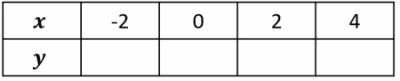Step 2: Find the values of $y$ for each $x$ value.

To work out the missing values, we use the equation like a formula, substituting the values from the table in, we get the following:

When $x = -2$, we get $y = (2\times-2) - 3 = -7$

When $x = 0$, we get $y = (2\times0) - 3 = -3$

When $x = 2$, we get $y = (2\times2) - 3 = 1$

When $x = 4$, we get $y = (2\times4) - 3 = 5$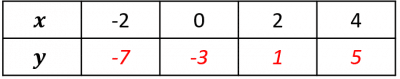Step 3: So, we know that the line passes through

$(-2, -7), (0, -3), (2, 1)$ and $(4, 5)$

Now all that remains is to plot them on a pair of axes and draw a straight line through them. The result should look like the graph below.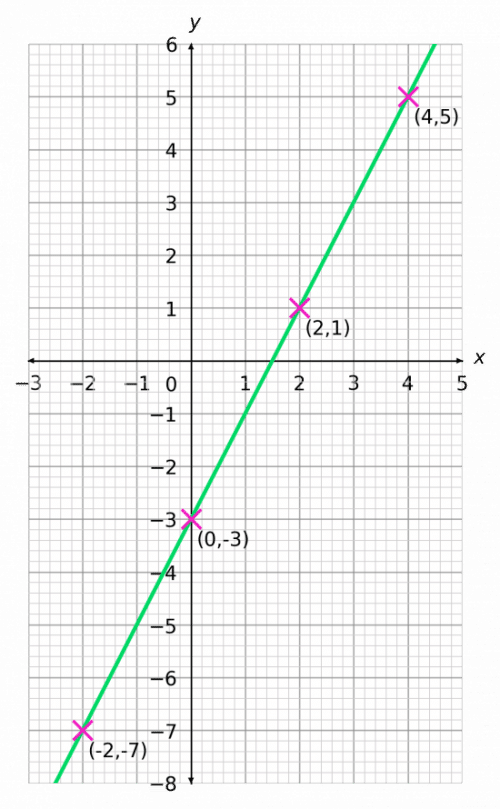Level 1-3GCSEKS3AQAEdexcelOCRWJECCambridge iGCSEEdexcel iGCSE

## Method 2: Using $y=mx+c$

You can use $y=mx+c$ to plot a straight line graph.

Example: Plot the straight-line graph with equation $3y + 9x = 12$.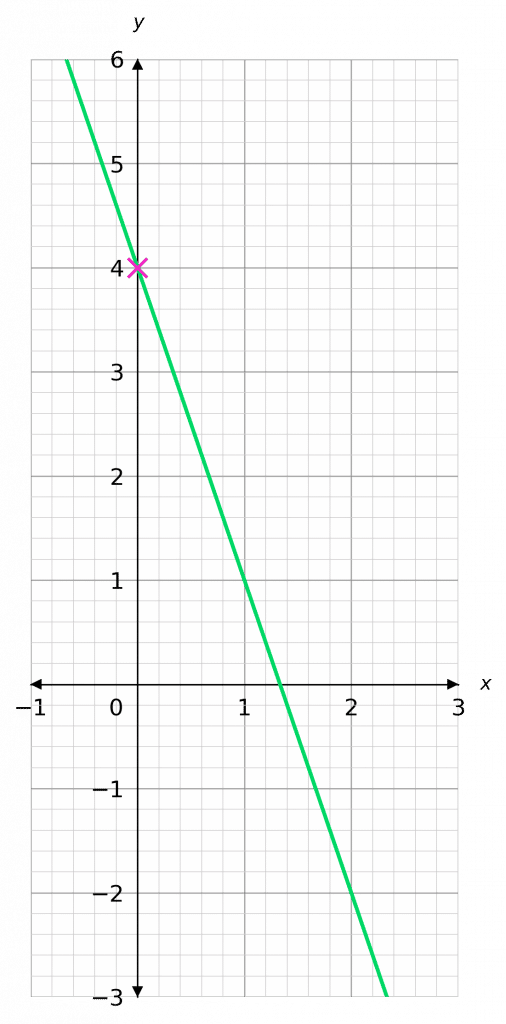Rearranging this equation to be in the form $y = mx +c$, by subtracting $9x$ from each side

$3y = -9x + 12$

Then, divide both sides by $3$, to get it in the form $y=mx+c$:

$y = -3x + 4$

So, the $y$-intercept is $4$, and the gradient is $-3$ – so each time $x$ increases by $1$, $y$ decreases by $3$

Level 4-5GCSEKS3AQAEdexcelOCRWJECCambridge iGCSEEdexcel iGCSE

## Drawing Straight Line Graphs Example Questions

To find the missing value, substitute the given values into the equation.

When $x = -1$, we get $y = \dfrac{1}{2} \times (-1) + 5 = 4.5$

When $x = 2$, we get $y = \dfrac{1}{2} \times (2) + 5 = 6$

When $y = 7$, we get $7 = \dfrac{1}{2}x + 5$

Subtract $5$ from both sides of this equation to get

$2 = \dfrac{1}{2}x$

Multiplying both sides by $2$, we immediately get $x = 4$. The completed table looks like:Plotting these points and using them to draw the graph should look like: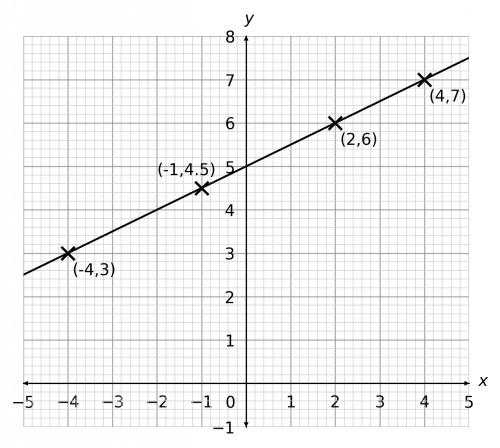Gold Standard Education

Let’s rearrange this equation. Subtract $1$ from both sides:

$2y = 8x - 1$

Then, divide both sides by $2$:

$y = 4x - \dfrac{1}{2}$

So, the $y$-intercept is $-\frac{1}{2}$, and the gradient is $4$ – so each time $x$ increases by $1$, $y$ increases by $4$.

This is enough information to draw the graph. The result should look like the figure below.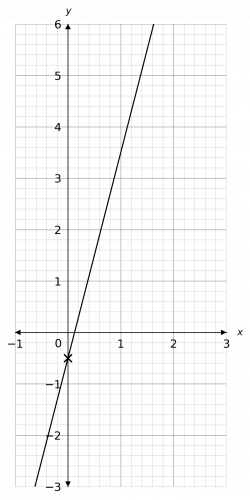Gold Standard Education

Rearranging this equation to be in the form $y = mx +c$, by adding $0.5$ to both sides,

$y = -0.5x + 0.5$

So, the $y$-intercept is $\dfrac{1}{2}$, and the gradient is $-\dfrac{1}{2}$ – so each time $x$ increases by $1$, $y$ decreases by $0.5$

The result should look like the figure below.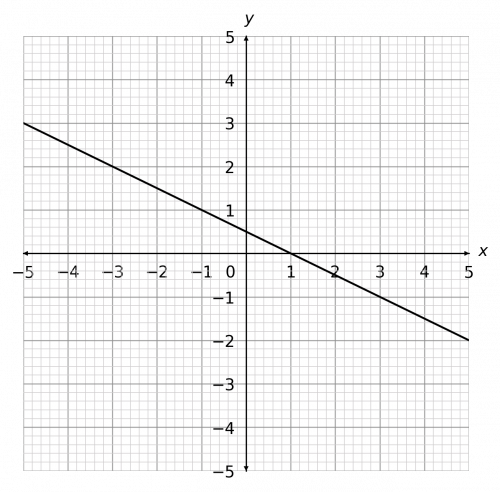Gold Standard Education

We can rearrange this equation by subtracting $2$ from both sides:

$2y = 3x - 2$

Then, dividing both sides by $2$:

$y = \dfrac{3}{2}x - 1$

So, the $y$-intercept is $-1$, and the gradient is $\dfrac{3}{2}$ – so each time $x$ increases by $1$, $y$ increases by $1.5$

The result should look like the figure below.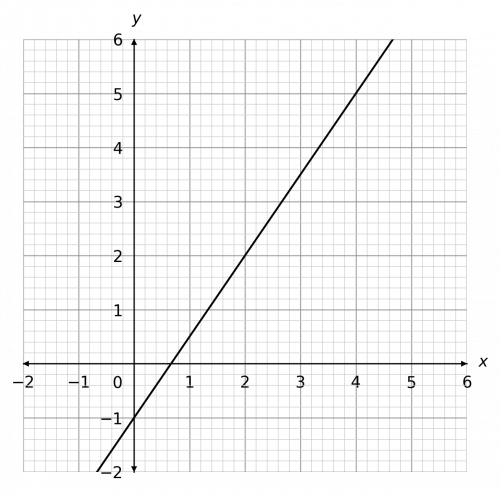Gold Standard Education

We can rearrange this equation by subtracting $4x \text{ and } 2$ from both sides:

$y = -4x - 2$

So, the $y$-intercept is $-2$, and the gradient is $-4$ – so each time $x$ increases by $1$, $y$ decreases by $4$

The result should look like the figure below.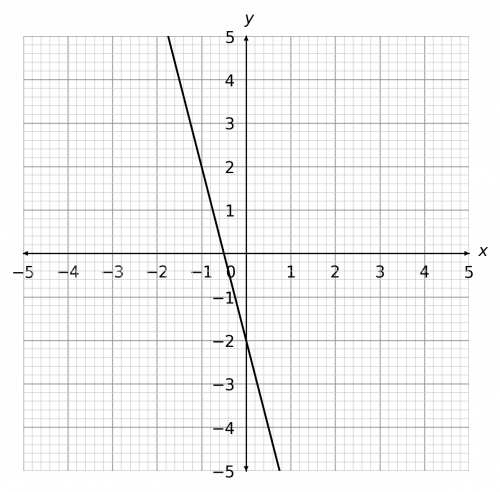Gold Standard Education

## Drawing Straight Line Graphs Worksheet and Example Questions

### (NEW) Drawing Straight Line Graphs Exam Style Questions - MME

Level 1-3GCSENewOfficial MME

## Drawing Straight Line Graphs Drill Questions

### Straight Line (2)

Level 1-3GCSEGold Standard Education

Level 4-5GCSEKS3

Level 4-5GCSEKS3